MORE IN Basic Electrical & Electronics Engg
MU First Year Engineering (Semester 1)
Basic Electrical & Electronics Engg
May 2015
Total marks: --
Total time: --
INSTRUCTIONS
(1) Assume appropriate data and state your reasons
(2) Marks are given to the right of every question
(3) Draw neat diagrams wherever necessary

1 (a) Convert the star circuit into its equivalent delta circuit.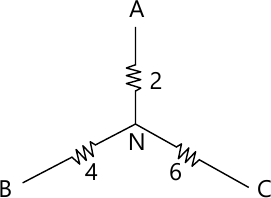3 M
1 (b) For the given circuit find the Norton equivalent between point A and B.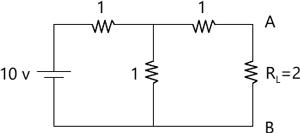3 M
1 (c) Draw an a.c. waveform indicate there on and explain (i) instantaneous value, (ii) peak value and (iii) time period for one cycle of the alternating quality.
3 M
1 (d) Differentiate between series and parallel resonance wth respect to
i) Impedance at resonance
ii) Current at resonance
iii) Magnification factor
3 M
1 (e) Draw the phasor diagram for 3 phase delta connected load with a lagging power factor. Indicate line and phase voltage and currents.
2 M
1 (f) Derive the emf equation for a single phase transformer.
4 M
1 (g) Draw a neat circuit diagram for a full wave bridge rectifier using 4 diodes and the corresponding inputs and output waveforms for voltage.
2 M

2 (a) Find the current through 1 ? resistance using Mesh Analysis.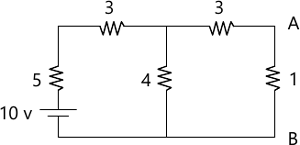6 M
2 (b) A coil having a resistance of 10? and an inductance of 40 mH is connected to a 200V, 50 Hz supply. Calculate the impedance of the coil, current, power factor and power consumed.
8 M
2 (c) Draw the phasor diagram of a transformer on no load and explain the various currents and voltage in it.
6 M

3 (a) Three similar coils each having a resistance of 10? and inductance of 0.04 H are connected in star across a 3 phase, 50Hz, 200V supply. Calculate the line current, total power absorbed, reactive volt amperes and total volt amperes.
8 M
3 (b) With the help of a neat diagram explain how short circuit test is conducted on a single phase transformer.
6 M
3 (c) Draw the circuit diagram of a full wave centre tapped rectifier with capacitor filter and the corresponding input and output waveforms.
2 M
3 (d) With the help of a neat diagram explain the output characteristics of an NPN transistor in common emitter configuration.
4 M

4 (a) Using source transformation find the current flowing through the 8? resistance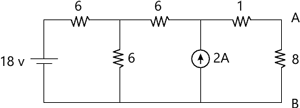7 M
4 (b) Find the rms value for the given waveform.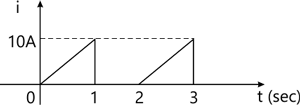5 M
4 (c) Two wattemeters are used to measure power in a 3? balanced star connected load using the two wattmeter method. The readings of the 2 wattmeters are 500W and 2500W respectively. Calculate the total power consumed by the 3? load and the power factor.
4 M
4 (d) With the help of a neat circuit diagram and input and output waveform explain the working of a half wave rectifier.
4 M

5 (a) Find the current through 8? resistance using Thevenins theorem.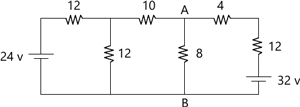8 M
5 (b) A resistance of 10? and a pure coil of inductance 31.8 mH are connected in parallel across 200V, 50 Hz supply. Find the total current and power factor.
4 M
5 (c) A 5KVA, 1000/200V, 50Hz, single phase transformer gave the following test results.
OC test (hv side): 1000V 0.24A 90W
SC test (hv) side: 50V 5A 110W
Calculate the equivalent circuit parameters of the transformer and draw the equivalent circuit diagram.
8 M

6 (a) Find the value of current flowing through the 5? resistance using superposition theorem.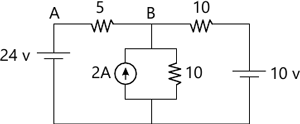7 M
6 (b) A series KLC circuit has the following parameter value: R=10?, L=0.014H, C=100?F Compute the resonant frequency, quality factor. Bandwidth, lower cut-off frequency and upper cut-off frequency.
7 M
6 (c) With the help of a neat circuit diagram and phasor diagram explain the 2-wattmeter method to measure power in a 3? balanced star connected load.
6 M

More question papers from Basic Electrical & Electronics Engg